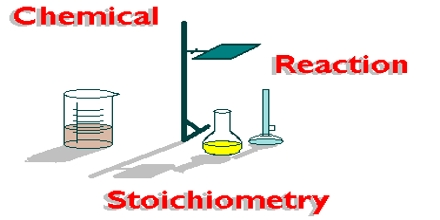# Stoichiometry of Electrolysis

Michael Faraday found that the mass of substances released at the electrodes is directly related to the total charge that has passed through the electrical circuit of the cell. To determine this we must know the current and the length of time it has been flowing, it is call stoichiometry of electrolysis.

Electric current is measured in amperes. An ampere (A) is the base SI unit of current equivalent to 1 coulomb/second. The quantity of electric charge passing through a circuit in a given amount of time is given by the following equation:

Electric charge (C) = electric current (C.s-1) x time (s)

Charge (C) = Current (A) x time (s)

In terms of symbols: Q = I x t

By definition, a current of one ampere flowing for one second is equal to one coulomb of charge. So measuring the current in a circuit and the time it flows for can give the amount of charge used.The charge on an electron is 1.6 x 10-19 coulombs. The charge carried by one mole of electrons will be:

6.02 x 1023 x 1.6 x 10-19 = 96,500 coulombs. We know that 96,500 C is also called 1 Faraday.

During electrolysis, the production of one mole of an element from its ion requires n x 96,500 C (where n is the charge on the ion).

For example, Na+ + e → Na will use one electron per ion. So this reaction will use a total of 96,500 C of charge. Similarly, Cu2+ + 2e → Cu will use two electrons per ion. So this reaction will use a total of 2 x 96,500 =193,000 C of charge.